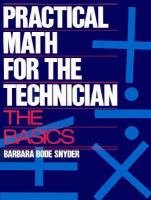Close

# Practical Math for the Technician the Basics

## by Barbara Bode-Snyder• ISBN: 9780132515139
• ISBN10: 013251513X

# Practical Math for the Technician the Basics

## by Barbara Bode-Snyder

• List Price: \$217.20
• Binding: Paperback
• Edition: 1
• Publisher: Pearson
• Publish date: 12/01/1990
• ISBN: 9780132515139
• ISBN10: 013251513X
used \$98.13
You save: 55%
Description: 1. Signed Whole Numbers Addition and Subtraction. 2. Signed Whole Numbers Multiplication and Division. 3. Order of Operations Please Excuse My Dear Aunt Sally. 4. Signed Decimal Numbers Addition, Subtration, Multiplication, Division. 5. Kirchoff's Laws. 6. Signed Fractions Different Types. 7. Signed Fractions Multiplication and Division. 8. Power in Watts Figuring your Utility Bill. 9. Signed FRactions Addition and Subtraction. 10. Conversion of FRactions to DEcimals to Fractions. 11. Magnitudess of Numbers Whole Numbers, Fractions, Decimals. 12. Percentages Tipping, Sales Tax, Range, Tolerance, Percent Difference. 13. Siginficant Digits and Rounding. 14. Powers of Ten. 15. Scientific Notation. 16. Prefixes. 17. Metric System. 18. Calculator Usage. 19. More Calculator usage. 20. Graphing. 21. Substituting Data into Formulas. 22. Electronics Applications. 23. Construction Applications. 24. Drafting Applications. 25. Number System for Computers. 26. Computer Applications. 27. Manipulating Equations. 28. Sets of Equations. 29. Solving Algebraic and Trig Equations. Index.
Expand description